# AP Board 9th Class Maths Notes Chapter 8 Quadrilaterals

Students can go through AP Board 9th Class Maths Notes Chapter 8 Quadrilaterals to understand and remember the concepts easily.

## AP State Board Syllabus 9th Class Maths Notes Chapter 8 Quadrilaterals

→ A quadrilateral is a simple closed figure bounded by four line segments.

→ The line segments joining any two opposite vertices are called diagonals.

→ The sum of the four interior angles of a quadrilateral is 360° or four right angles.

→ A quadrilateral in which one pair of opposite sides are parallel is called a trapezium.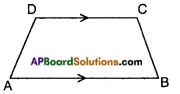→ A quadrilateral in which both pairs of opposite sides are parallel is called a parallelogram.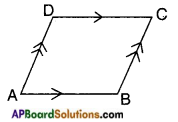→ A parallelogram in which the adjacent sides are equal is called a rhombus.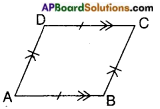→ A parallelogram in which one angle is right angle is called a rectangle.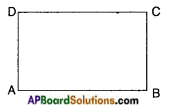→ A parallelogram in which adjacent sides are equal and one angle is right angle is called a square.→ A quadrilateral in which the two pairs of adjacent sides are equal is called a ‘kite’.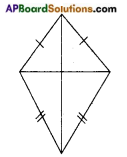→ In, a parallelogram

• diagonals bisect each other.
• opposite angles are equal.
• both pairs of opposite sides are equal.
• diagonal divides the parallelogram into two congruent triangles.

→ In a rhombus, diagonals bisect each other at right angles and the diagonals are unequal.

→ In a square diagonals are equal and bisect each other at right angles.

→ In a rectangle diagonals are equal and bisect each other.→ The line segment joining the mid points of two side of a triangle is parallel to third side and also half of it.

→ The line drawn through the mid point of one side of a triangle and parallel to another side will bisect the third side.

→ The intercepts made by the transversal on three or more parallel lines are equal to one another.